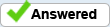# LiveCycle: Is it possible to copy the results on a calculation from one field to another?

My form has lists of entries that are subtotaled. I want each subtotal to display in separate field of a list of subtotals, resulting in a total for the form.
The subtotal field has a calculate event in FormCalc:
if (exists(Row.numAmount) == 1) then
Sum(Row[*].numAmount)
endif
I added an exit event in JavaScript:
form1.SubformY.Subform4.Table9.Row5.Total.rawValue = this.rawValue;

Can a field have more than one event?
Is there a conflict between FormCalc and JavaScript?
What would be a better way?
Thanks!
VC

Vicky Curran

Fist of all, if you have a calculated field this field should be read only and you should not add a script to the exit event of a calculated field.
Considering that the Subtotal fields are already calculated (and read only) you can also add a script to the calculate event in the Total field to sum the Subtotals.

Q: Can a field have more than one event?
A: You can add scripts to different events in the same field, to enter and exit events, for example, since they make sense. If you have a calculated field it should be read only and should have a script only in the calculate event. In other words, a field can have more than one script in different events, depending on the situation.

Q: Is there a conflict between FormCalc and JavaScript?
A: You have to choose between FormCalc and JavaScript when you add a script to a specific event in the field, but you can use FormCalc for an event and JavaScript for another one in the same field. What is impossible is to use FormCalc and JavaScript in the same event.

Q: What would be a better way?
A: There isn't one answer. It depends on your knowledge, preferences and resources you want to use. I prefer to use JavaScript because you can do more things with it, such as to create functions, and because it is the same language (its core) used for Acro Forms and Acrobat automation. But some operations are easier to implement with FormCalc, such as totaling the values in a column in a table with a varying number or rows.

By Almir R V Santos

" First of all, if you have a calculated field this field should be read only and you should not add a script to the exit event of a calculated field. Considering that the Subtotal fields are already calculated (and read only) you can also add a script to the calculate event in the Total field to sum the Subtotals."

Thanks for figuring out why the script was not working. However, I need to display the subtotals in another field before totalling them, like this:
Subtotal1: xxxx
Subtotal 2: xxxx
Total costs: XXXXX
How can I copy the subtotal from the calculated/read only field into the new field and use the amount to calculate the total?

Vicky Curran

You can "copy the subtotal from the calculated/read only field into the new field" in two ways:

1) In the calculate event of the new field you can add a script like this, considering that Subtotal1 is in the same Subform as the new field:
JavaScript:
`this.rawValue = Subtotal1.rawValue;`

FormCalc:
`this.rawValue = Subtotal1.rawValue`

2) If you use the same name for the Subtotal field and the new field you can set the Data Binding of the first one to "Use global data" and all the fields with that name will show the same value. You have to be careful with this procedure to avoid conflicts with fields with the same name in other subforms.Almir R V Santos